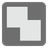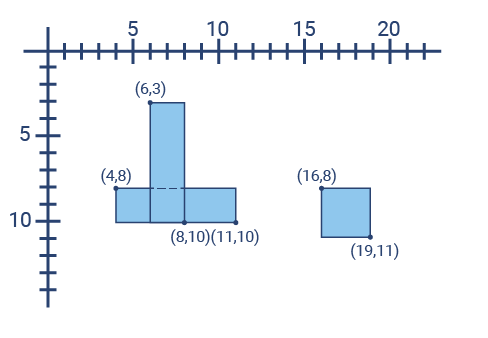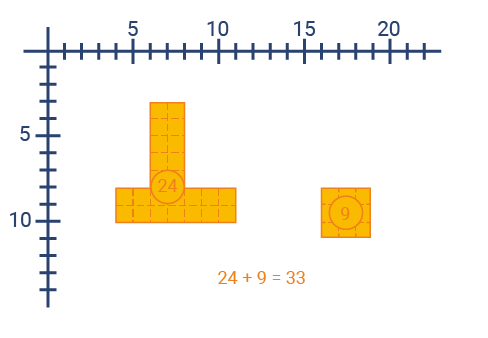Rectangles Union
Elementary+
English

Your mission is to calculate the area covered by a union of rectangles. The rectangles can have a non-empty intersection, which means that a simple sum of given rectangle areas doesn't work. Every rectangle is represented as 4 integers. The first two integers are the coordinates of a left-top corner, and the next two - of a bottom right corner.Input: Iterable with tuples.

Output: Int.

Example:

```rectangles_union([
(6, 3, 8, 10),
(4, 8, 11, 10),
(16, 8, 19, 11)
]) == 33
```